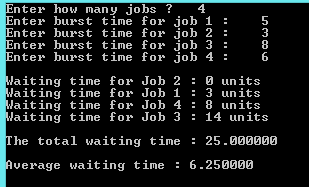## Sunday, 28 December 2014

### CPU Scheduling algorithm - SJF

 This is a C program which implements one of the CPU Scheduling algorithm called Shortest Job First(SJF). Shortest Job First algorithm reduces the waiting time but it is impossible to implement as the processor must know all the jobs/processes in advance.
PROGRAM :

```#include <stdio.h>
#include <stdlib.h>

int main()
{
int i,j,temp,temp2,n,bt,wt,a;
float avgWt,totalWt=0;
wt=0;
printf("Enter how many jobs ?\t");
scanf("%d",&n);
for(i=1;i<=n;i++)
{
printf("Enter burst time for job %d :\t",i);
scanf("%d",&bt[i]);
a[i]=i; //stores job has how much burst  time in array  i
}
for(i=1;i<=n;i++)  // ascending order of burst times and a[i]

for(j=i;j<=n;j++)

if(bt[i] > bt[j]){
temp=bt[i];
bt[i]=bt[j];
bt[j]=temp;
temp2=a[i];
a[i]=a[j];
a[j]=temp2;
}

printf("\nWaiting time for Job %d : 0 units \t",a);
for(i=2;i<=n;i++)
{
wt[i]=bt[i-1]+wt[i-1];
printf("\nWaiting time for Job %d : %d units \t",a[i],wt[i]);
totalWt = totalWt + wt[i];
}
printf("\n\nThe total waiting time : %f\t",totalWt);
avgWt= totalWt/n;
printf("\n\nAverage waiting time : %f\t\n\n",avgWt);
return 0;

}
```
OUTPUT :C - CPU Scheduling algorithm(SJF)